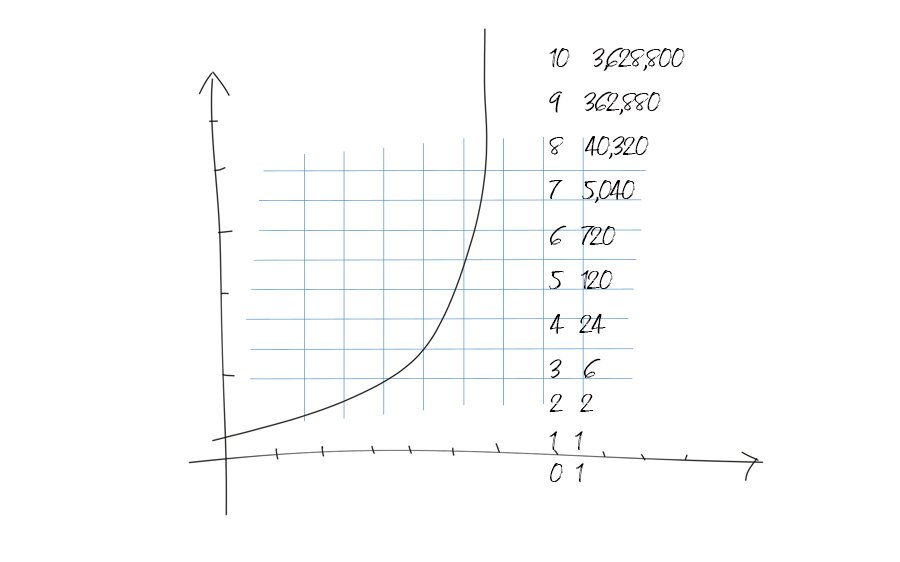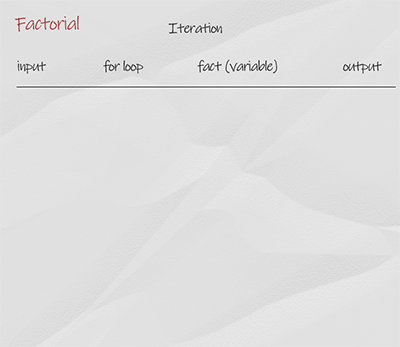The factorial of a number, denoted as n!, is the product of all integers between n and 1. n! = n x (n-1) x (n-2) … x1. The value of 0! is 1. You can use iteration and recursion in implementation.### Iteration

In iteration implementation, you assign the initial value of fact to be 1. This is default value for number 0 and 1. Then you use for loop iterate from 2 to the input number. Each time fact is updated to previous fact times the loop variable i. When it finishes, return fact.

## Doodle### Recursion

In recursion implementation, the function calls itself with input number minus 1. The base condition is when the input number is 0, function returns 1. Then the call stack returns. The recursion simplifies the problem conceptually, but is not as efficient as loop-based approach.

## Doodle